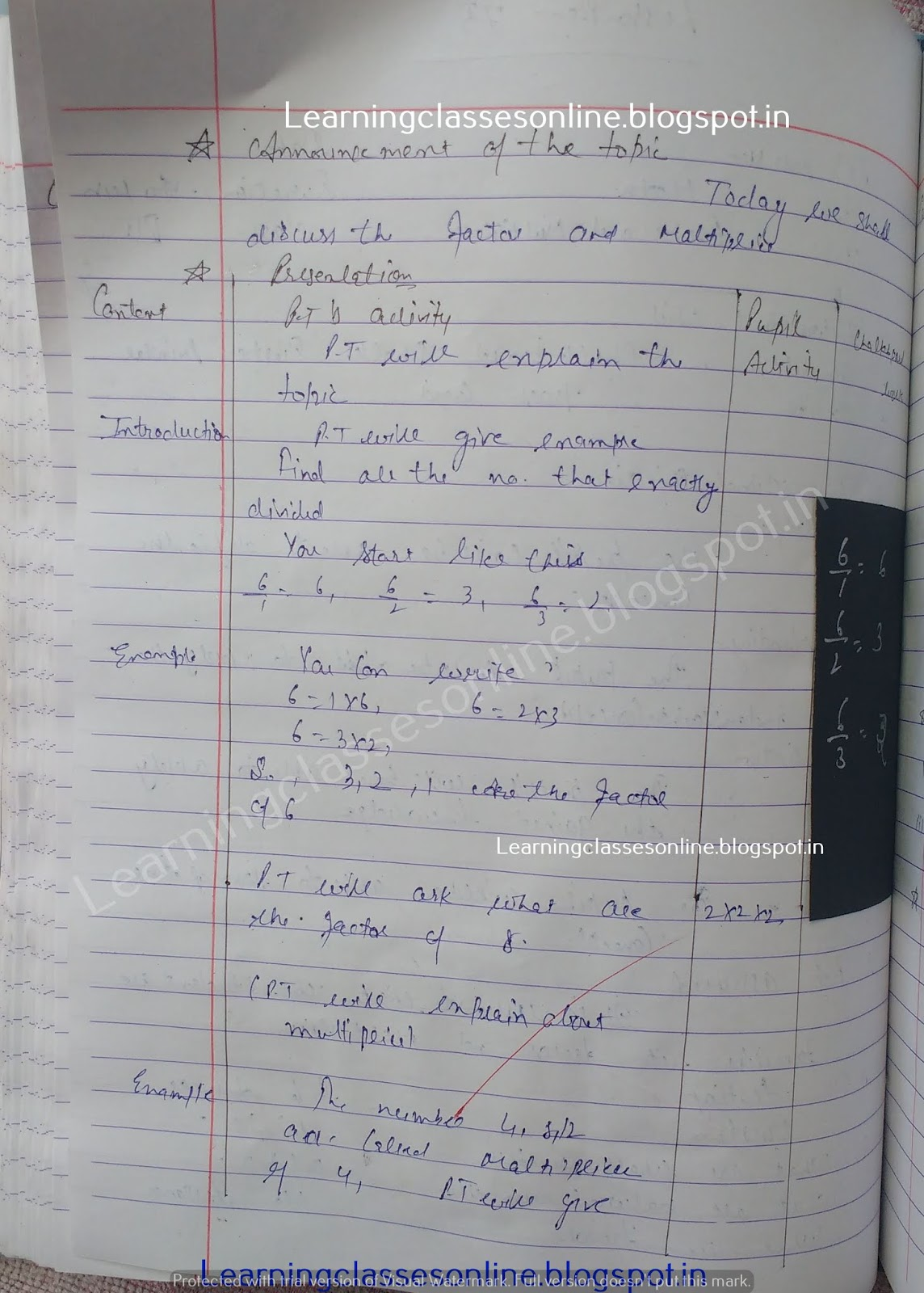Math Mega Teaching Lesson Plan on factor and multiply -->

# Math Mega Teaching Lesson Plan on factor and multiply

Math Mega Teaching Lesson Plan on factor and multiply for grade 5, 6 and 7 school teachers.

Note: The Mathematics Lesson Plan given below is just an example. You can change the Name, Class, Course, Date, Duration, etc. according to your needs.